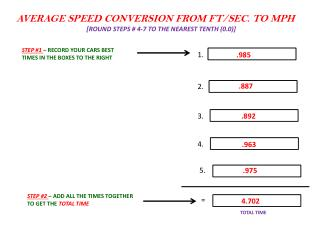DownloadDownload PresentationAVERAGE SPEED CONVERSION FROM FT/SEC. TO MPH [ ROUND STEPS # 4-7 TO THE NEAREST TENTH (0.0)]

# AVERAGE SPEED CONVERSION FROM FT/SEC. TO MPH [ ROUND STEPS # 4-7 TO THE NEAREST TENTH (0.0)]

Télécharger la présentation## AVERAGE SPEED CONVERSION FROM FT/SEC. TO MPH [ ROUND STEPS # 4-7 TO THE NEAREST TENTH (0.0)]

- - - - - - - - - - - - - - - - - - - - - - - - - - - E N D - - - - - - - - - - - - - - - - - - - - - - - - - - -
##### Presentation Transcript

1. AVERAGE SPEED CONVERSION FROM FT/SEC. TO MPH [ROUND STEPS # 4-7 TO THE NEAREST TENTH (0.0)] STEP #1 – RECORD YOUR CARS BEST TIMES IN THE BOXES TO THE RIGHT 1. .985 .887 2. .892 3. 4. .963 5. .975 STEP #2 – ADD ALL THE TIMES TOGETHER TO GET THE TOTAL TIME = 4.702 TOTAL TIME

2. (4.702 ÷ 5) STEP #3 – DIVIDE THE TOTAL TIME BY THE NUMBER OF RACES TO FIND THE AVERAGE TIME .9404 AVERAGE TIME (ROUND STEPS #4-7 TO NEAREST TENTHS) STEP #4 – DIVIDE THE TRACK LENGTH (32’) BY THE AVERAGE TIME TO FIND THE AVERAGE FT/SEC 34.0 AVERAGE FT/SEC STEP #5 – MULTIPLY THE AVERAGE FT/SEC BY 60 TO FIND THE AVERAGE FT/MIN 2040 AVERAGE FT/MIN STEP #6 – – MULTIPLY THE AVERAGE FT/MIN BY 60 TO FIND THE AVERAGE FT/HOUR 122400 AVERAGE FT/HOUR STEP #7 – DIVIDE THE AVERAGE FT/HOUR BY 5280(THE NUMBER OF FT IN A MILE) TO FIND THE AVERAGE MPH 23.2 AVERAGE MPH

3. CALCULATING ACCELERATION OR a = Vf – Vi/t

4. CALCULATING FORCE m = 39.6g a = 91.7 ft/ 1. F = m · a (m = lowest mass) & (a = acceleration) Mass MUST be in kg NOT g (divide mass by 1000) 2. 39.6g ÷ 1000 = .0396kg 3. F = .0396 kg · 91.7 ft/ 4. F = 3.63132 kg·ft/ or 3.6N